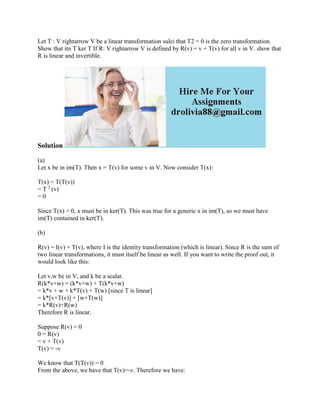Successfully reported this slideshow.

# Let T - V rightarrow V be a linear transformation sulci that T2 -.docx×

# Let T - V rightarrow V be a linear transformation sulci that T2 -.docx

Let T : V rightarrow V be a linear transformation sulci that T2 = 0 is the zero transformation. Show that itn T ker T If R: V rightarrow V is defined by R(v) = v + T(v) for all v in V. show that R is linear and invertible.
Solution
(a)
Let x be in im(T). Then x = T(v) for some v in V. Now consider T(x):

T(x) = T(T(v))
= T 2 (v)
= 0
Since T(x) = 0, x must be in ker(T). This was true for a generic x in im(T), so we must have im(T) contained in ker(T).
(b)
R(v) = I(v) + T(v), where I is the identity transformation (which is linear). Since R is the sum of two linear transformations, it must itself be linear as well. If you want to write the proof out, it would look like this:
Let v,w be in V, and k be a scalar.
R(k*v+w) = (k*v+w) + T(k*v+w)
= k*v + w + k*T(v) + T(w) [since T is linear]
= k*[v+T(v)] + [w+T(w)]
= k*R(v)+R(w)
Therefore R is linear.
Suppose R(v) = 0
0 = R(v)
= v + T(v)
T(v) = -v
We know that T(T(v)) = 0
From the above, we have that T(v)=-v. Therefore we have:
T(-v) = 0
-T(v) = 0 [T is linear]

Once again we know that T(v)=-v. Therefore we have:
-(-v) = 0
v = 0
Therefore R(v)=0 --> v=0
Since R is linear, this means R is invertible.
.

Let T : V rightarrow V be a linear transformation sulci that T2 = 0 is the zero transformation. Show that itn T ker T If R: V rightarrow V is defined by R(v) = v + T(v) for all v in V. show that R is linear and invertible.
Solution
(a)
Let x be in im(T). Then x = T(v) for some v in V. Now consider T(x):

T(x) = T(T(v))
= T 2 (v)
= 0
Since T(x) = 0, x must be in ker(T). This was true for a generic x in im(T), so we must have im(T) contained in ker(T).
(b)
R(v) = I(v) + T(v), where I is the identity transformation (which is linear). Since R is the sum of two linear transformations, it must itself be linear as well. If you want to write the proof out, it would look like this:
Let v,w be in V, and k be a scalar.
R(k*v+w) = (k*v+w) + T(k*v+w)
= k*v + w + k*T(v) + T(w) [since T is linear]
= k*[v+T(v)] + [w+T(w)]
= k*R(v)+R(w)
Therefore R is linear.
Suppose R(v) = 0
0 = R(v)
= v + T(v)
T(v) = -v
We know that T(T(v)) = 0
From the above, we have that T(v)=-v. Therefore we have:
T(-v) = 0
-T(v) = 0 [T is linear]

Once again we know that T(v)=-v. Therefore we have:
-(-v) = 0
v = 0
Therefore R(v)=0 --> v=0
Since R is linear, this means R is invertible.
.